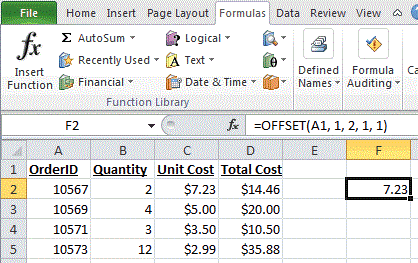## Excel Office

Excel How Tos, Tutorials, Tips & Tricks, Shortcuts

# How to use Excel OFFSET function

This Excel tutorial explains how to use the OFFSET function with syntax and examples.

## Excel OFFSET function Description

The Microsoft Excel OFFSET function returns a reference to a range that is offset a number of rows and columns from another range or cell.

OFFSET function is a built-in function in Excel that is categorized as a Lookup/Reference Function. The OFFSET function can be entered as part of a formula in a cell of a worksheet.

Worked Example:   Sum through n months in ExcelExplanation: Based on the example above, the following OFFSET function return:

```=OFFSET(A1, 1, 2, 1, 1)
Result: reference to cell C2   (and thus would display \$7.23)

=OFFSET(C3, -1, -2, 1, 1)
Result: reference to cell A2   (and thus would display 10567)```

## Syntax

The syntax for the OFFSET function in Microsoft Excel is:

`OFFSET( range, rows, columns, [height], [width] )`

### Arguments

range
The starting range from which the offset will be applied.
rows
The number of rows to apply as the offset to the range. This can be a positive or negative number.
columns
The number of columns to apply as the offset to the range. This can be a positive or negative number.
height
Optional. It is the number of rows that you want the returned range to be. If this parameter is omitted, it is assumed to be the height of range.
width
Optional. It is the number of columns that you want the returned range to be. If this parameter is omitted, it is assumed to be the width of range.